# How to Generate Random Values by a List in Different Cases in Excel

Sometimes we may create some fake data for testing or some other purpose. If we want to create an amount of numbers, we may feel it is annoying by entering random numbers one by one. For this instance, we can use RANDBETWEEN function to create a lot of numbers between a certain range quickly. On the other side, sometimes we may want to create random values refer to a given list, for example select some random numbers from a given number list, you can also select objects by given list as well. This article will show you the way to generate random values, and also generate values from a given list.

## Method 1: Generate Random Numbers by RANDBETWEEN Function

Step 1: Select a range for saving the random numbers. Select a blank cell as the first cell in your selected range. Then enter the formula =RANDBETWEEN(1,100). In this case, we will generate numbers between 1 and 100.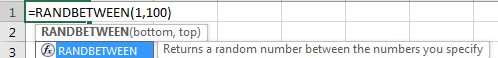Step 2: Press Enter. We get a random number 57. Just between our specified numbers.Step 3: Drag the fill handle to fill the selected range. Now we create a range of random data. You may notice that the first cell value is changed. That’s because the formula is applied to other cells so after releasing your mouse to complete the task, it was recalculated.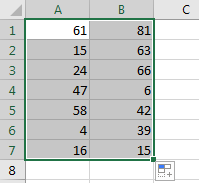## Method 2: Generate Random Numbers by a Given List

Prepare a list with fruit.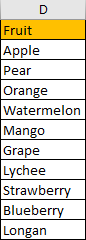Step 1: Select a blank cell, enter the formula =INDEX(\$D\$2:\$D\$11, RANDBETWEEN(2, 11)). In this formula \$D\$2:\$D\$11 is the list area, 2 is the first row number for the area, and 11 is the last row number for the area. You can change parameters per your demands.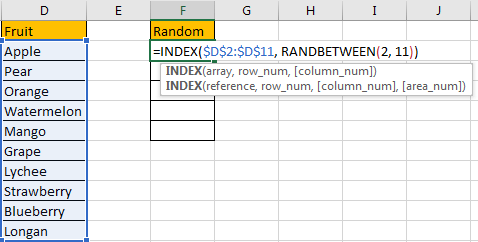Step 2: Press Enter to check result. Verify that the random value is generated properly and it is also listed in original fruit list.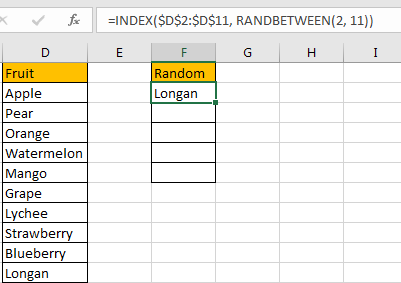x
How to Remove Line Breaks Carriage Returns in Excel

Step 3: Drag the fill handle down till F6. Verify that fruits are generated by random.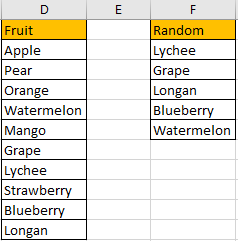NOTICE:

1. In this step, when dragging fill handle to fill other cells, some errors may occur, for example:
2.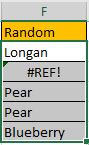You just need to double click on error cell and re-apply the formula.

1. Sometimes the random values may be duplicate. See above screenshot, ‘Pear’ are displayed twice.

## Method 3: Generate Random Numbers by a Given List Between Certain Range

Prepare a list with numbers. If we want to generate random values from this list and at the same time between number 1 to 50, how can we do? Let’s see steps below.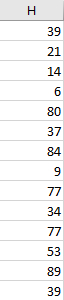Step 1: Select a blank cell, enter the formula =INDEX(\$A\$1:\$A\$14, RANDBETWEEN(1,50)). Then press Enter to check if the generated number matches the two criteria.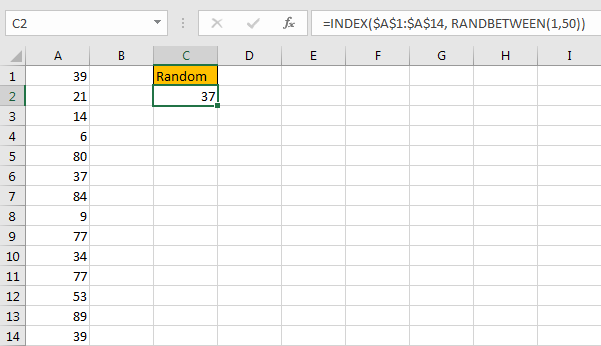Obviously, number 37 is between range 1 to 50, and it is also listed in original list.

### Related Functions

• Excel INDEX function
The Excel INDEX function returns a value from a table based on the index (row number and column number)The INDEX function is a build-in function in Microsoft Excel and it is categorized as a Lookup and Reference Function.The syntax of the INDEX function is as below:= INDEX (array, row_num,[column_num])…
• Excel RANDBETWEEN Function
The Excel RANDBETWEEN function returns a random integer number that is between the numbers you specify.The syntax of the RANDBETWEEN function is as below:= RANDBETWEEN (bottom,top)….

Related Posts

Approximate Match with Multiple Criteria by INDEX & MATCH

In Excel, INDEX function and MATCH function are often used together for returning data from specific position. And MATCH function is one of Excel lookup & reference functions that can return approximate value by setting match type. Above all, through ...

How to Find the First or Last Positive or Negative Number in a Column/List in Excel

Suppose we have a list of data entry, we want to look up the first positive number among them, is there any way to find it out? And if we want to look up the first or last negative number, ...

How to Create Dynamical Drop-Down List and Sort by Alphabetical Order in Excel

In our daily work we may need to create a dynamical dropdown list and sort all values by alphabetical order. To create a dropdown list like this, we need to apply some built-in features like ‘Define Name’ and ‘Data Validation’ ...

How to Average Absolute Values in Excel

We can use AVERAGE function to calculate average of certain values. We can use ABS function to get absolute values for both positive number and negative number. If we want to get the average absolute values, we need to combine ...

How to Look Up the Lowest Value in A List by VLOOKUP/INDEX/MATCH Functions in Excel

VLOOKUP function is very useful in our daily work and we can use it to look up match value in a range, then get proper returned value (the returned value may be just adjacent to the match value). Sometimes we ...

How to Dynamically Extract Unique Values from A Column List in Excel

Suppose we have a list of some objects in one column, and some of them are duplicate, they also can be replaced by typing different object name, here’s the question, how can we dynamically extract unique values from this list ...

How to Find the Earliest and Latest Date in Excel

We have a range of dates and we want to look up the earliest and the latest date based on certain criteria like the earliest date for a showing movie, we can use MIN and MAX functions with IF function ...

How to Create Dynamic Drop Down List without Blank in Excel

This post will guide you how to create dynamic drop down list without blank cells in Microsoft Excel. In Excel, and you can use Data Validation feature to improve the efficiency of data entry in excel, and it also be ...

How to Stack Data from Multiple Columns into One Column in Excel

In previous article, I have shown you the method to split data from one long column to multiple columns by VBA and Index function. This time if we want to stack data from multiple columns to one column, how can ...

How To Align Duplicate Values within Two Columns in Excel

This post will guide you how to align duplicate values within two columns based on the first column in your worksheet in Excel. How do I use an formula to align two columns duplicate values in Excel. Aligning Duplicate Values ...

Sidebar